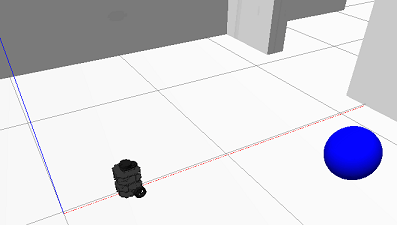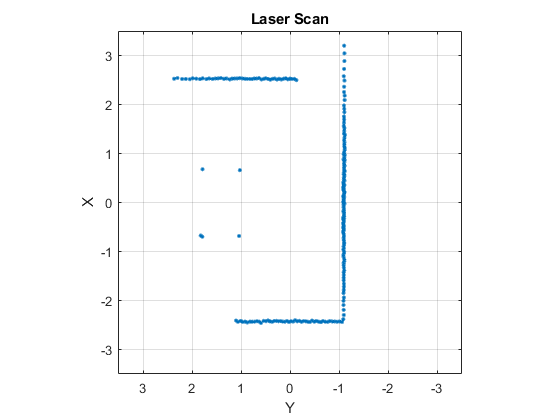# Communicate with the TurtleBot

This example introduces the TurtleBot® platform and the ways in which MATLAB® users can interact with it. Specifically, the code in this example demonstrates how to publish messages to the TurtleBot (such as velocities) and how to subscribe to topics that the TurtleBot publishes (such as odometry).

The TurtleBot must be running for this example to work.

### Connect to the TurtleBot

The TurtleBot must be running. If you are using a real TurtleBot and followed the hardware setup steps in Get Started with a Real TurtleBot, the robot is running. If you are using a TurtleBot in simulation and followed the setup steps in Get Started with Gazebo and Simulated TurtleBot, launch one of the Gazebo® worlds from the desktop (`Gazebo Office`, for instance).

In your MATLAB instance on the host computer, run the following command. Replace `ipaddress` with the IP address of the TurtleBot. This line initializes ROS and connects to the TurtleBot.

```ipaddress = "http://192.168.178.133:11311"; rosinit(ipaddress)```
```Initializing global node /matlab_global_node_88195 with NodeURI http://192.168.178.1:59934/ ```

If the network you are using to connect to the TurtleBot is not your default network adapter, you can manually specify the IP address of the adapter that is used to connect to the robot. This might happen if you use a Wireless network, but also have an active Ethernet connection. Replace `IP_OF_TURTLEBOT` with the IP address of the TurtleBot and `IP_OF_HOST_COMPUTER` with the IP address of the host adapter that is used to connect to the robot:

```rosinit("IP_OF_TURTLEBOT","NodeHost","IP_OF_HOST_COMPUTER"); ```

Display all the available ROS topics using:

```rostopic list ```

If you do not see any topics, then the network has not been set up properly. Refer to the beginning of this document for network setup steps.

### Move the Robot

You can control the movement of the TurtleBot by publishing a message to the `/cmd_vel` topic. The message has to be of type `geometry_msgs/Twist`, which contains data specifying desired linear and angular velocities. The TurtleBot's movements can be controlled through two different values: the linear velocity along the X-axis controls forward and backward motion and the angular velocity around the Z-axis controls the rotation speed of the robot base.

Set a variable `velocity` to use for a brief TurtleBot movement.

`velocity = 0.1; % meters per second`

Create a publisher for the `/cmd_vel` topic and the corresponding message containing the velocity values. Use the publisher with structure format messages for better performance.

```robotCmd = rospublisher("/cmd_vel","DataFormat","struct") ; velMsg = rosmessage(robotCmd);```

Set the forward velocity (along the X-axis) of the robot based on the `velocity` variable and publish the command to the robot. Let it move for a moment, and then bring it to a stop.

```velMsg.Linear.X = velocity; send(robotCmd,velMsg) pause(4) velMsg.Linear.X = 0; send(robotCmd,velMsg)```To view the type of the message published by the velocity topic, execute the following:

`rostopic type /cmd_vel`
```geometry_msgs/Twist ```

The topic expects messages of type `geometry_msgs/Twist`, which is exactly the type of the `velMsg` created above.

To view which nodes are publishing and subscribing to a given topic, use the command: `rostopic`` info TOPICNAME`. The following command lists the publishers and subscribers for the velocity topic. MATLAB is listed as one of the publishers.

` rostopic info /cmd_vel`
```Type: geometry_msgs/Twist Publishers: * /matlab_global_node_88195 (http://192.168.178.1:59934/) Subscribers: * /gazebo (http://192.168.178.133:39227/) ```

### Receive Robot Position and Orientation

The TurtleBot uses the `/odom` topic to publish its current position and orientation (collectively denoted as pose). Since the TurtleBot is not equipped with a GPS system, the pose will be relative to the pose that the robot had when it was first turned on.

Create a subscriber for the odometry messages

`odomSub = rossubscriber("/odom","DataFormat","struct");`

Wait for the subscriber to return data, then extract the data and assign it to variables `x`, `y`, and `z`:

```odomMsg = receive(odomSub,3); pose = odomMsg.Pose.Pose; x = pose.Position.X; y = pose.Position.Y; z = pose.Position.Z;```

Note: If you see an error, then it is likely that the `receive` command timed out. Make sure that odometry is being published and that your network is set up properly.

Display the x, y, and z values

`[x y z]`
```ans = 1×3 -2.6176 1.0007 -0.0010 ```

The orientation of the TurtleBot is stored as a quaternion in the `Orientation` property of `pose`. Use `quat2eul` (Robotics System Toolbox) to convert into the more convenient representation of Euler angles. To display the current orientation, `theta`, of the robot in degrees, execute the following lines.

```quat = pose.Orientation; angles = quat2eul([quat.W quat.X quat.Y quat.Z]); theta = rad2deg(angles(1))```
```theta = 0.1556 ```

Subscribe to the lidar topic:

`lidarSub = rossubscriber("/scan","DataFormat","struct");`

After subscribing to the lidar topic, wait for the data and then display it with rosPlot.

```scanMsg = receive(lidarSub); figure rosPlot(scanMsg)```To continuously display updating lidar scans while the robot turns for a short duration, use the following while loop:

```velMsg.Angular.Z = velocity; send(robotCmd,velMsg) tic while toc < 20 scanMsg = receive(lidarSub); rosPlot(scanMsg) end``````velMsg.Angular.Z = 0; send(robotCmd,velMsg)```

### Disconnect from the Robot

Clear the workspace of publishers, subscribers, and other ROS-related objects when you are finished with them.

`clear`

Use `rosshutdown` once you are done working with the ROS network. Shut down the global node and disconnect from the TurtleBot.

`rosshutdown`
```Shutting down global node /matlab_global_node_88195 with NodeURI http://192.168.178.1:59934/ ```

### Next Steps

Refer to the next example: Explore Basic Behavior of the TurtleBot